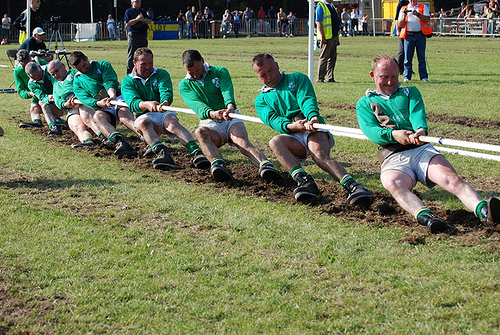# The Tug of WarIn a tug of war, each of the two teams apply 1000N force at the ends of a rope, which is found to be in equilibrium, the tension in the rope is -

$Assumptions-$ Assume the rope to be ideal.

Give the answer without the unit of force.

×